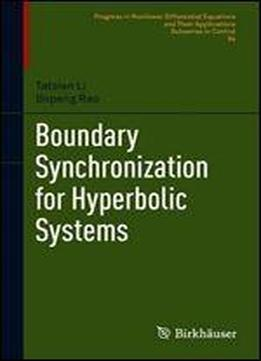# Boundary Synchronization For Hyperbolic Systems (progress In Nonlinear Differential Equations And Their Applications) by Tatsien Li / 2020 / English / PDF

Within this carefully presented monograph, the authors extend the universal phenomenon of synchronization from finite-dimensional dynamical systems of ordinary differential equations (ODEs) to infinite-dimensional dynamical systems of partial differential equations (PDEs). By combining synchronization with controllability, they introduce the study of synchronization to the field of control and add new perspectives to the investigation of synchronization for systems of PDEs. With a focus on synchronization for a coupled system of wave equations, the text is divided into three parts corresponding to Dirichlet, Neumann, and coupled Robin boundary controls. Each part is then subdivided into chapters detailing exact boundary synchronization and approximate boundary synchronization, respectively. The core intention is to give artificial intervention to the evolution of state variables through appropriate boundary controls for realizing the synchronization in a finite time, creating a novel viewpoint into the investigation of synchronization for systems of partial differential equations, and revealing some essentially dissimilar characteristics from systems of ordinary differential equations. Primarily aimed at researchers and graduate students of applied mathematics and applied sciences, this text will particularly appeal to those interested in applied PDEs and control theory for distributed parameter systems.

views: 129